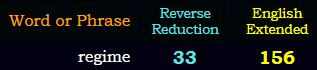## Cipher## Apr 10, 2017

### CNN - removing Assad from power is a priority - 911/227 Nikki Haley connections

http://edition.cnn.com/2017/04/09/middleeast/syria-missile-strike-chemical-attack-aftermath/index.html
This is a huge article so I'm just gonna show some connections to Nikki Haley and Assad
Now their "plan" is to remove Assad from power.. this is getting better and better by the dayNikki Haley connects to Bashar al-Assad in the most incredible way, and it's very obvious why they're coming out with this story today

First note that Assad is 51 years old at the moment
Nikki Haley = 5+9+2+2+9 + 8+1+3+5+7 = 51 (Single Reduction)
Haley = 8+1+12+5+25 = 51 (English Ordinal)
al-Assad = 8+6 + 8+8+8+8+5 = 51 (Reverse Reduction)
From the release of this story to Assad's birthday is a span of 5 months and 1 day...She's born on 20th of January and this story is coming 9 months and 11 days before her next birthday, note that Assad is born on 9/11...In the title we see Syria regime = 100+700+90+9+1 + 90+5+7+9+40+5 = 1056 (English Extended)Nikki Haley is 29th Ambassador to the United Nations
Ambassador = 27+13+2+1+19+19+1+4+15+18 = 119 (Francis Bacon)

Measuring from Haley's birthday to Assad's, we find very interesting numbers, 234 days (7 months 22 days) .. you just can't escape Pi coding with these people..chemical weapons attack = 24+19+22+14+18+24+26+15 + 4+22+26+11+12+13+8 + 26+7+7+26+24+16 = 364 (Reverse Ordinal)
364 could also stand for a full circle - one year = 60+50+5 + 700+5+1+90 = 911 (English Extended)It's extremely interesting that we have 234 coding and they are saying that chemical attack killed 89 people
If you add up 89 decimal places of Pi you will get to 432 (234 and 432 are actually connected to the Fibonacci sequence and this overlap happening with digits of Pi is very important, but I'm gonna save that for another post)
chemical weapons attack = 3+8+5+30+9+3+1+20 + 900+5+1+60+50+40+90 + 1+100+100+1+3+10 = 1440 (Jewish)
The attack took place in Khan Sheikhoun = 11+8+1+14 + 19+8+5+9+11+8+15+21+14 = 144 (English Ordinal)
Assad = 1+19+19+1+4 = 44 (English Ordinal)
Forty four = 6+15+18+20+25 + 6+15+21+18 = 144 (English Ordinal)

Assad regime = 1+90+90+1+4 + 80+5+7+9+30+5 = 322 (Jewish)
US government responded with "Tomahawk Cruise missiles" = 7+12+14+26+19+26+4+16 + 24+9+6+18+8+22 + 14+18+8+8+18+15+22+8 = 322 (Reverse Ordinal)

Word "regime" has very interesting gematria156th Fibonacci number has 33 digits ;)
Link to first 300 Fibonacci numbers - http://www.maths.surrey.ac.uk/hosted-sites/R.Knott/Fibonacci/fibtable.html